﻿ Magnetism
﻿

## Magnetic Flux Through The Open Surface S

B: magnitude of the magnetic field (T, tesla), α: angle between B and perpendicular line to surface S.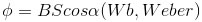## Magnetic Forces On Moving Charges

v: velocity of the charge (m/s)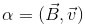## Force On A Current Carrying-Wire

I: current (A), l: length of the wire (m)## Force Between Two Parallel Current-Carrying Wire

r: distance between two wires (m)## Magnetic Field Of A Long Current Charge Wire

r: perpendicular distance from the center of the wire (m)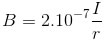## Magnetic Field Of A Loop

N: numbers of turns per unit length of solenoid (turns/m)## Induced Electromotive Force- Faraday's Law

N: numbers of turns per loop (turns/m)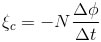## Inductance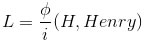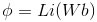## Electric Energy## Magnetic Energy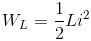## Electric-Magnetic Energy